# Divisor power sum function

(diff) ← Older revision | Latest revision (diff) | Newer revision → (diff)
Jump to: navigation, search
This article defines an arithmetic function or number-theoretic function: a function from the natural numbers to a ring (usually, the ring of integers, rational numbers, real numbers, or complex numbers).
View a complete list of arithmetic functions

## Definition

Letbe a real number (typically an integer). The divisor power sum function (sometimes called the divisor function)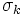is defined as the following arithmetic function from the natural numbers to the real numbers: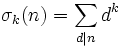.

The sum is over all the positive divisors of.

### Definition in terms of Dirichlet product

The divisor power sum function is defined as: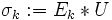.

Here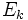is thepower function, andis the all ones function.

## Particular cases

### Thecase

The casegives the divisor count function, i.e., the function that counts the number of positive divisors of.

### The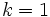case

The casegives the divisor sum function, i.e., the sum of all the positive divisors.

## Dirichlet series

Further information: Formula for Dirichlet series of divisor power sum function

The Dirichlet series foris given by: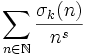.

This is related to the Riemann zeta-function by the following identity, that holds both formally and for the corresponding meromorphic functions: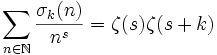.